# Advanced Multiplication Worksheets: Large Numbers

Advanced multiplication worksheets are exclusively available for students of grade 3 through grade 6. Their speed and accuracy in multiplying two whole numbers is greatly improved by working on these pdf worksheets. The exercises incorporate practice problems and word problems involving 3-digit, 4-digit and 5-digit multiplicands and single-digit, two-digit, and three-digit multipliers. Now, you can download some of these worksheets for free.

Multiplying numbers with more zeros

Practice on these printable worksheets to end confusion on multiplication of numbers ending with zeros.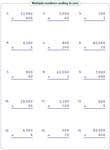Multiplying 3 or 4 digits by single-digit

Multiply three-digit or four-digit numbers by a single-digit number to find the product in each problem.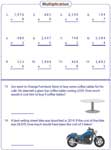Standard:

With word problems:

Multiplying 3-digit by 2-digit

Work on these advanced multiplication worksheets where 3-digit multiplies 2-digit.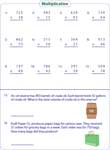Standard:

With word problems:

Multiplying 4 or 5 digit by 2-digit

Multiply 4-digit or 5-digit with 2-digit numbers to solve each problem. Real-life problems are included.Standard:

With word problems:

Multiplying 3-digit by 3-digit

Long multiplication problems where 3-digit multiplies 3-digit. The numbers to be multiplied are vertically arranged.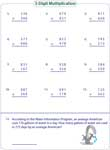Standard:

With word problems:

Multiplying 4 or 5 digit by 3-digit

Build your skills on multiplication. This section has 15 standard problems with / without word problems.Standard:

With word problems:

Multiplying Large Numbers: Standard

Long Multiplication gets tougher! Try solving these problems with large number multiplicands. All the worksheets have only vertical standard problems.By 1-digit:

By 2-digit:

By 3-digit:

Multiplying Large Numbers with Word Problems

Practice multiplying 100 thousands, millions and 10 millions by 1, 2 or 3-digit numbers. Word problems are included in each pdf worksheet.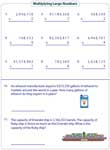By 1-digit:

By 2-digit:

By 3-digit:

Related Worksheets